# Find an equation of best fit in Geogebra

/, PreCalculus, Statistics/Find an equation of best fit in Geogebra

## Find an equation of best fit in Geogebra

1. Go to Geogebra.org.
2. Click on Geogebra Math Apps.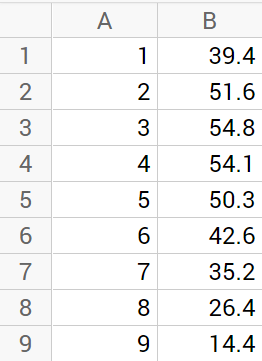5. Click in the graph area, then right click and choose Graphics.
6. In the Basic tab, change your x- and y- mins and maxes to represent your data points.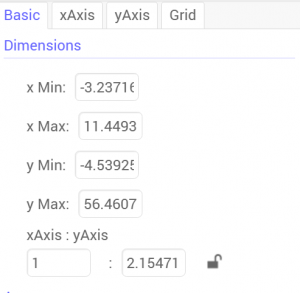7. Click on the Grid tab and Show Grid if you want. Then click the X to close the properties dialog box.
8. Highlight your data in the spreadsheet, then right click on it.
9. Choose Create > List of Points.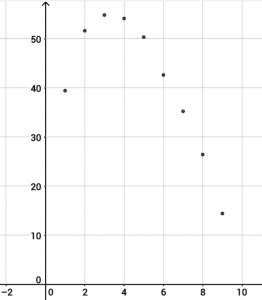10. Click on the Menu icon in the upper right and choose View and check Input Bar.
11. The Input Bar appears in the bottom of your screen. Type y=a*(x-h)^2 + k and press Enter. When a dialog box pops up, click Create Sliders.
12. Click on a slider and choose the Set label style button to show the Name and Value.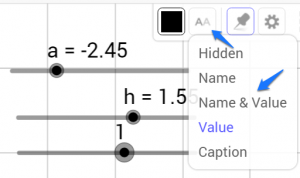13. Right click on a slider and choose Object Properties. Adjust the Min: and Max: and Increment: for each of your three sliders to reflect your data.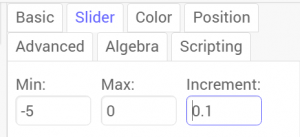15. To see how your equation compares to the one Geogebra calculates, select the data in the spreadsheet.
16. Choose the table – bar graph icon. Choose Two Variable Regression Analysis.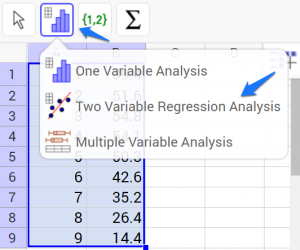17. The data from this table most closely represents a quadratic so choose Polynomial.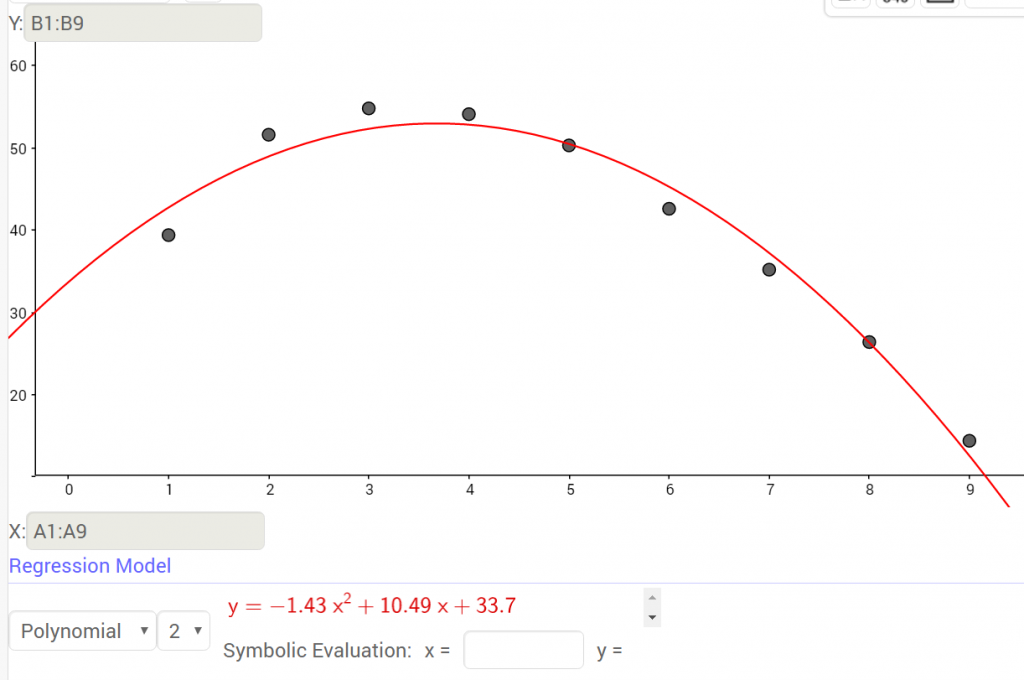18. How did the equation you created using the sliders compare to the Geogebra Regression Model?
By | 2017-11-13T22:01:55+00:00 September 24th, 2016|Graphing Calculator, PreCalculus, Statistics|0 Comments

### About the Author: Stacie Bender